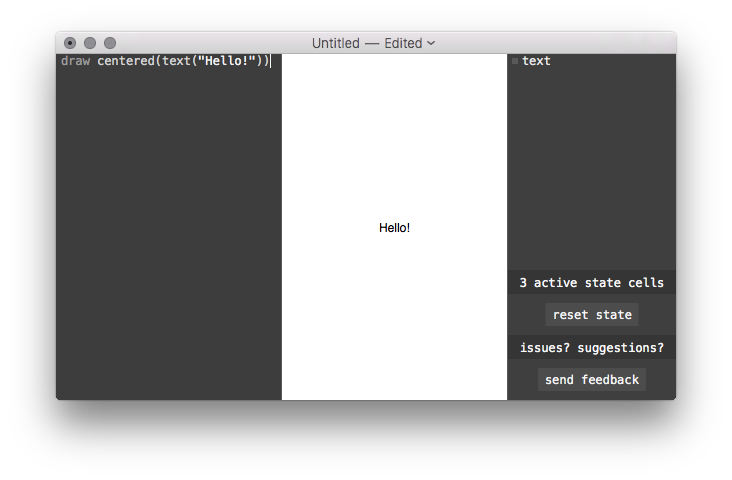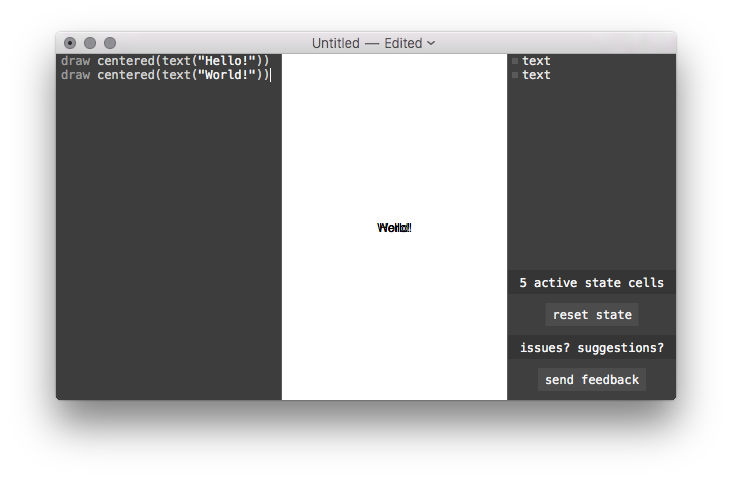# DisplayScript

## Basic Concepts

DisplayScript is a text-based programming environment. Interface elements are placed according to a textual program which appears on the left hand side of the editor. Here's a program that displays the text, "Hello!"Programs (and parts of programs) will usually appear in these reference pages as preformatted text instead of as a screenshot. For example, the above program might appear as

`draw centered(text("Hello!"))`

DisplayScript programs are run from top to bottom, line by line. Adding more draw lines will add more elements to your interface:

```draw centered(text("Hello!"))
draw centered(text("World!"))```

Here's what that looks like:Beautiful!

Each of these lines is called a statement. Normally, each new line will start a new statement. Blank lines are OK: they just do nothing. See Line Breaks and Statements for the full rules about line breaks.

Two forward slashes // will mark the rest of the line as a comment. Comments are ignored while running the program, so you can write whatever you want in them. Usually comments are used to write helpful notes:

```// Center the text so it looks good.
draw centered(text("Hello!"))```

Let's take a closer look at our draw statement. The keyword draw tells us what kind of statement this is. It's followed by some text describing what should be drawn:

`centered(text("Hello!"))`

This text is called an expression. Expressions produce values: in this case, the expression produces a "drawable" value. There are other kinds of expressions, too, that produce different kinds of values:

```// Math expressions:
1 + 2
sqrt(17)

// Strings:
"eleven"

// Comparisons:
5 > 2

// Arrays:
[1, 3, 1 + 3]```

You can inspect the value of any expression using show.

```// Math expressions:
show(1 + 2)
show(sqrt(17))

// Strings:
show("eleven")

// Comparisons:
show(5 > 2)

// Arrays:
show([1, 3, 1 + 3])```

You can see shown values in the tree view on the right side of the editor. Using show is a great way to see what your program is doing.

Expressions can be nested in other expressions and grouped using parentheses:

`show((1 + 2) * 3 > 7 * count([1, 1]))`

Check out Types of Values for all the types of values and expressions that are available.

### Naming Values

The var statement creates a named binding from a value.

```var speed = 9
var time = 0.4
var distance = speed * time
show(distance)```

Using a binding in an expression will yield its value. After you've created the binding, you can assign a new value to it using an = statement.

```var string = "Hello!"
draw centered(text(string))

string = "World!"
draw centered(text(string))```

Just like you can name values with var, you can name sequences of statements using function:

```function showTwoThings(a, b) {
show(a)
show(b)
}
showTwoThings(1, 2)
showTwoThings("x", 1 > 2)```

You can run the statements in a function by calling it using parentheses. Values in the parentheses are passed into the function as arguments. If a function returns a value, you can use it in expressions:

```function square(a) {
return a * a
}
show(square(3) - square(2))```

Everything you've seen called like this is a function: show, text, centered, sqrt and so on. In fact, functions are values themselves:

```show(text)
show(sqrt)
show(show)```

See the page on Functions and Drawables for more about functions.

Drawables represent interface elements. Like functions, they are a sequence of statements, but they don't take any arguments or return anything. You can use draw on a drawable to run its statements.

```drawable hello {
draw centered(text("Hello!"))
}
draw hello```

You can also apply functions to drawables. One important function is at, which positions a drawable on the screen:

```drawable hello {
draw fill(gray(0.7))
draw centered(text("Hello!"))
}
draw at(10, 10, 100, 100, hello)```

There are many other functions that take and return drawables. As we've seen, text returns a drawable that draws its argument as text (see Text Rendering for more about text). The centered function centers a drawable. You can see a full list of these drawable functions in the Drawable Function Index.

You can also measure a drawable to see how big it is.

```var hello = text("Hello!")
var size = measure(0, 0, hello)
show(size.width)
show(size.height)```

Calling measure with a proposed width and height returns a record with the width and height of the drawable. The page on Measurement and Layout goes into more detail.

### Basic Control Flow

Control flow statements change the flow of execution. The if statement, for example, only runs the statements in braces if a condition holds:

```if 1 < 2 {
show("Looks like 1 is less than 2.")
}```

The for statement runs the statements in braces once for each element in an array.

```var y = 0
for name in ["Joe", "Sally"] {
draw at(0, y, 100, 100, centered(text(name)))
y = y + 100
}```

See Control Flow for more about if, for, and the other control flow statements.

### State

When your program runs, it starts from a clean slate: all the numbers, functions, drawables, and so on are created as their statements are run. State variables let you pass values from one run to the next.

```state color = gray(0.5)
function modifyColor(dx, dy) {
next color = {
r: color.r + dx / 500,
g: color.g,
b: color.b + dy / 500
}
}
draw dragHandler(function (x, y) {}, modifyColor, function () {})```

Note how we use next color to update the color rather than just assigning to it directly. State variables aren't changed until the next time the code is run. Check out State and Identity for more about how state works.